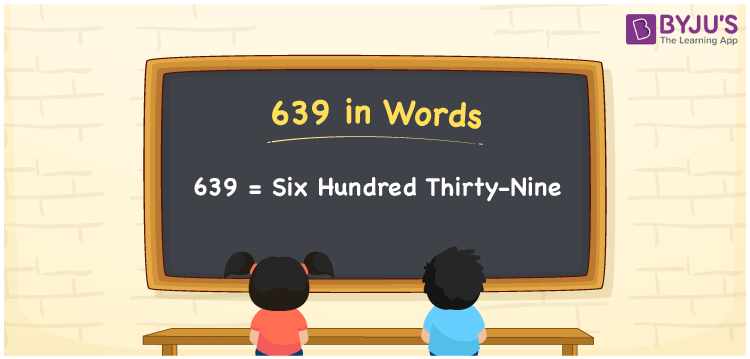# 639 in Words

639 in words is written as Six hundred thirty-nine. In both the International System of Numerals and the Indian System of Numerals, 639 is written as Six hundred thirty-nine. The number 639 is a Cardinal Number as it represents some quantity. For example, “there are 639 tourists”.

 639 in Words Six hundred thirty-nine Six hundred thirty-nine in Number 639

## 639 in English Words

We write 639 in English Words using the letters of the English alphabet. Therefore, we read 639 in English as “Six hundred thirty-nine.”## How to Write 639 in Words?

To write 639 in words, we shall use the place value chart. In the place value chart, write 6 in the hundreds, 3 in the tens, and 9 in the ones, respectively. Now let us make a place value chart to write the number 639 in words.

 Hundreds Tens Ones 6 3 9

Thus, we can write the expanded form as

6 × Hundred + 3 × Ten + 9 × One

= 6 × 100 + 3 × 10 + 9 × 1

= 600 + 30 + 9

= 639

= Six hundred thirty-nine.

639 is a natural number, the successor of 638 and the predecessor of 640.

639 in words – Six hundred thirty-nine

• Is 639 an odd number? – Yes
• Is 639 an even number? – No
• Is 639 a perfect square number? – No
• Is 639 a perfect cube number? – No
• Is 639 a prime number? – No
• Is 639 a composite number? – Yes

## Frequently Asked Questions on 639 in Words

Q1

### How to write 639 in words?

639 in words is written as Six hundred thirty-nine.
Q2

### How to write 639 in the International and Indian System of Numerals?

In both, the system of numerals, 639 in words, is written as Six hundred thirty-nine.
Q3

### What is the preceding number of 639?

The number that precedes 639 is 638.